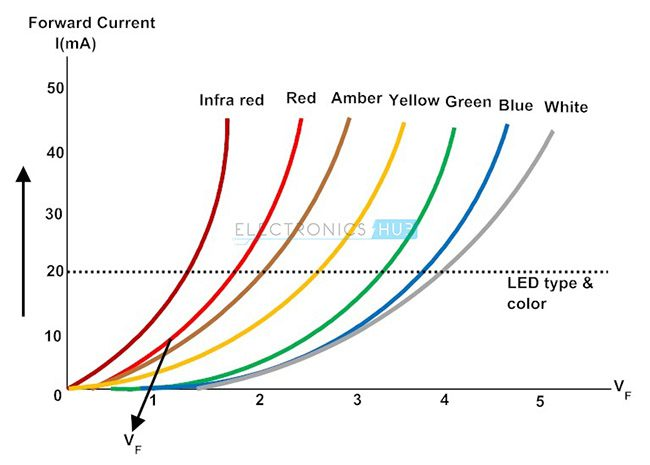Objectives:

• To be able to describe an experiment to obtain the I–V characteristics of a filament lamp and a Diode;
• To be able to describe the uses and benefits of using a Diode.

I-V characteristics

I-V characteristics were introduced in the previous page when introducing Ohm’s law. They are graphical characteristics showing how the current (I) varies as the potential difference (V) across the component varies. The current is always plotted on the y-axis of a graph and the voltage on the x-axis.

Previously the I-V characteristic of a resistor (or wire) at a constant temperature was mentioned, many electrical components have varying temperatures and therefore their resistances vary which would affect the current-voltage relationship.

I-V characteristics of a filament lampNotice the old style bulb circuit symbol

If the variable resistor is altered so as to change the overall resistance in the circuit, both the voltmeter and ammeter readings will also change.

If a set of results were taken whereby both positive and negative readings of voltage and current were collected the following current-voltage graph can be drawn.This graph displays non-linear characteristics, therefore the component does not obey Ohm’s law. This makes sense as Ohm’s law is only relevant to components at constant temperatures.

For a filament bulb, as the voltage increases across it the greater the temperature becomes resulting in increased vibrations of the ions, this in term reduces the magnitude of the rise in current. At low voltages, e.g. 0 V, any increase in p.d. results in a large increase in current, with a greater voltage, an increase results in a smaller rise in current.

Similar to the the resistor, or wire, at a constant temperature, a filament bulb shows the same characteristics in the negative quadrant (with the ratio of p.d. to current varying with the same pattern).

I-V characteristics of a diodeIf the variable resistor is altered so as to change the overall resistance in the circuit, both the voltmeter and ammeter readings will also change.

If a set of results were taken whereby both positive and negative readings of p.d. and current were collected the following current-voltage graph can be drawn.This graph displays non-linear characteristics, therefore the component does not obey Ohm’s law. This makes sense as Ohm’s law is only relevant to components at constant temperatures.

Diodes appear peculiar components and often confuse students when first experimenting with them, due to the 0 V and 0 A readings shown with the current in one direction. However this is the purpose of a diode, they only allow current to flow in one direction.

Diodes are designed such that the potential difference across it needs to be at a minimum of 0.6 V before any current can pass through it. With as the p.d. rises beyond 0.6 V, the current begins to rise rapidly  as can be seen in the graph above.

The following points are important to understand and note;

• Between 0 and 0.6 V: The resistance is extremely high (infinite), so no current can flow ($R = \infty \ \Omega$ ,$I = 0 \ A$ )
• 0.6 V – 0.7 V (approximately): The resistance decreases, the current can increase     ($R = \ \downarrow \Omega$ ,$I = \ \uparrow A$ )
• 0.7 V and upwards: The resistance decreases further, the current increases even more ($R = \ \Downarrow \Omega$ ,$I = \ \Uparrow A$ )
• With any negative p.d: The resistance is extremely high (infinite), so no current can flow ($R = \infty \ \Omega$ ,$I = 0 \ A$ )

Extension: LED I-V characteristics

An LED is a light emitting diode, the have very similar characteristics to standard diodes. A key point to note however is that the threshold voltage (the minimum voltage required for a current to flow) varies depending on the colour (this will become more apparent when studying quantum physics later in the year). The following helps to show this (but with limited detail):